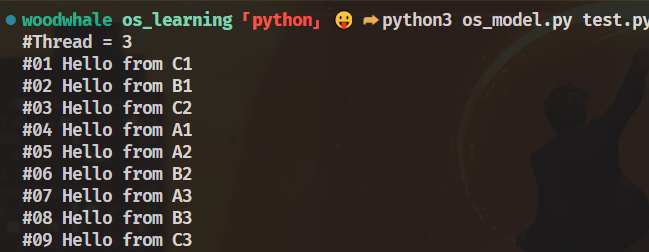# 【OS】Python模拟简单的操作系统

## Background

• 应用程序
• 系统调用
• 操作系统的内部实现

• 真实的操作系统
• 编译内核环境
• qemu模拟

• 应用程序 == Python Code + Syscall
• 操作系统 == Python Code + 假想的IO设备

``````def main():
sys_write("Hello Python OS!")
``````

## Core

• write（输出字符）
• spawn（创建一个状态）
• choose（随机选择一个状态）
• sched（随机切换到某个状态并且执行）

``````def sys_write(msg):
print(msg)

def sys_choose(x):
return randmo.choice(x)

def sys_spawn(x):
running.append(x)   # 状态队列
``````

• 保存`当前状态`
• 恢复`目的状态`

Python的生成器支持`send`方法，可以让生成器变为双向通道。`send`可以把参数发送给生成器，同时让这个参数成为上一条yield表达式的求值结果，并且将生成器推进到下一条yield表达式，然后把yield右边的值返回给send方法的调用者。

## Code

``````import sys, random

def __init__(self, func, *args) -> None:
self.func = func(*args)
self.ret = None

def step(self):
syscall, args, *_ = self.func.send(self.ret)
self.ret = None
return syscall, args

class OS:
SYSCALLS = ("choose", "write", "spawn", "sched")

def __init__(self, src) -> None:
vars = {}
exec(src, vars)
self.main = vars["main"]

def run(self):
while running:
try:
match (t := running).step():
case "choose", x:  # choose this and exec
t.ret = random.choice(x)
case "write", msg:
print(msg, end="")
case "spawn", (fn, args):
case "sched", _:
random.shuffle(running)
except StopIteration:
running.remove(t)
random.shuffle(running)

if __name__ == "__main__":
if sys.argv.__len__() < 2:
print("python3 os_model.py [src]")
sys.exit(0)

for syscall in OS.SYSCALLS:
src = src.replace(f"sys_{syscall}", f"yield '{syscall}', ")
OS(src).run()
``````

• 需要传入一个参数，指定需要执行的python文件
• 读取这个python文件的代码，将sys_write这类的syscall函数转为`yield`构造的生成器函数
• 使用OS类进行执行，OS在`__init__`的时候使用`exec`载入了python文件的信息
• 执行run函数，在每个线程的step函数执行后，执行不同的syscall函数
• 维护`running`线程队列
• 每次执行`sys_spawn`的时候会将这个函数加入到`running`队列
• 一个线程执行结束抛出`StopIteration`的异常，同时从`running`队列删除，在打乱线程队列

`python3 os_model.py test.py`

test.py如下：

``````count = 0

def Tprint(name):
global count
for i in range(3):
count += 1
sys_write(f'#{count:02} Hello from {name}{i+1}\n')
sys_sched()

def main():
n = sys_choose([3, 4, 5])
for name in 'ABCDE'[:n]:
sys_spawn(Tprint, name)
sys_sched()
``````• sys_sched只能由用户手动调用，而真实OS会被动调用
• 只有一个终端 ，同时没有stdin的输入
• 没有实现fork等较常用的syscall的操作

codebox/mosaic: Python script for creating photomosaic images (github.com)

## Idea

• 程序被建模为高级语言 (Python) 的执行和系统调用
• 系统调用的实现未必一定需要基于真实或模拟的计算机硬件
• 操作系统的 “行为模型” 更容易理解